﻿ Logger Pro for the Observation of Single-Slit Diffraction in the Determination of Metal Coefficients of Linear Thermal ExpansionPublications are Open
Access in this journal
Article Versions
Export Article
• Normal Style
• MLA Style
• APA Style
• Chicago Style
Research Article
Open Access Peer-reviewed

### Logger Pro for the Observation of Single-Slit Diffraction in the Determination of Metal Coefficients of Linear Thermal Expansion

International Journal of Physics. 2021, 9(4), 218-221. DOI: 10.12691/ijp-9-4-5
Received June 02, 2021; Revised July 05, 2021; Accepted July 22, 2021

### Abstract

Experiments using Logger Pro-aided observation of single-slit diffraction patterns have been performed to determine coefficients of linear thermal expansion of metals. The sample metals were iron and brass. The calculation involves weighted linear regression analysis of 1/z against temperature, where z is the distance between the end to the center of the central bright line, and a computer-aided χ2 goodness of fit test. From the χ2 goodness of fit test, it is concluded that the relation between 1/z and temperature is linear, in agreement with theory. The coefficients of linear thermal expansion for iron obtained agree well with the reference value, while that for brass is close to the reference values.

### 1. Introduction

For more than a century, the study of thermal expansion of solids has remained an interesting subject in materials science and its applications 1. While many recent studies have been focussed on the development of metal alloys with specific properties including zero and negative coefficients of thermal expansion 2, 3, 4, 5, the basic measurement methods remain of interest, especially for didactic purposes.

The fractional change in length of a metal rod due to a change in temperature,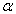is generally very small, therefore various methods have been developed to enable a more precise measurement of the change in length than a direct measurement. A good example is the laser single-slit diffraction method developed by Fakhruddin 6. Another example is the optical lever method developed by Inbanathan et al 7.

Meanwhile, advances in information technology have brought various sensors and software which can aid in collecting, recording, sharing, and analyzing the dynamics of physical quantities. A good example is Logger Pro, a general-purpose data collection and analysis computer program, which can be downloaded at http://www.vernier.com. It has found many applications in physics and other related fields for the automatic measurement, recording, demonstration and analysis of various physical phenomena 8.

In the single-slit diffraction method developed by Fakhruddin 6, a metal rod was submerged in a water bath, and the temperature of the bar was approximated by the water temperature as measured by a mercury thermometer. The calculation involves a weightless linear regression analysis of 1/z against temperature, where zis the distance between the end to the center of the central bright line. This paper reports the application of Logger Pro to automate the data collection in the single-slit diffraction for the determination of coefficients of linear thermal expansion of metals in the temperature range of 30-90°C. The final calculation of the coefficient of linear thermal expansion involves the results of a weighted linear regression analysis. To justify the assumption of the linear equation of 1/z against temperature, a computer-aided χ2 goodness of fit test 9 was also performed. The objectives of this study are to develop an inexpensive apparatus for the determination of coefficients of linear thermal expansion of metals using the single-slit diffraction method suitable for an undergraduate physics laboratory and to test the validity of the theoretical model.

The sample metals were iron and brass. These metals were chosen since they are inexpensive and easily available. Iron represents a pure metal, while brass represents an alloy.

• Figure 1. The experimental setup

### 2. Experimental Design

The experimental setup is shown in Figure 1. A metal rod of the material to be investigated is submerged in a water bath containing an electrical heating element. The diffraction slit consists of two razor blades separated at a narrow distance, making a slit of width w. A light beam is emitted at a right angle to the diffraction slit, producing a diffraction pattern on a screen. When heated, the metal rod will expand, causing the slit width and the fringe distance z in the diffraction pattern to increase. A temperature sensor is attached to the metal rod, and a LabPro interface reads the temperature data as a function of time and sends them to a Logger Pro display on a laptop.

Suppose initially the metal rod has a length L0 and temperature T0. After the metal rod is heated to a temperature T, its length increases to L and the slit width is increased accordingly, and it follows that 6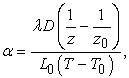(1)

where λ is the light wavelength, D is the distance between the screen and the slit, and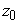is the initial value of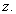Equation (1) can be rewritten in a linear form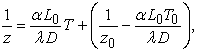(2)

or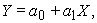(3)

where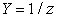and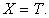Therefore a weighted linear regression of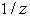against temperature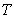can be performed to determine the coefficients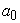and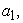as well as their errors, and to calculate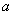and its error from these quantities. Given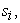the measurement error of each measured value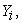then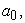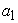and their errors can be obtained using a weighted linear regression analysis through the following equations 10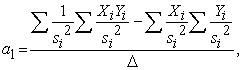(4.a)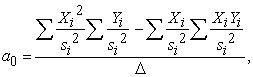(4.b)

where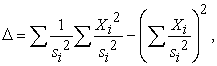(5)

and their errors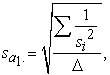(6.a)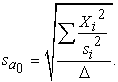(6.b)

From Equations (2) and (3) it is seen that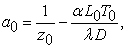(7)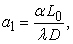(8)

thereforeand its error can be calculated from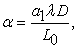(9.a)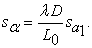(9.b)

Whereas the coefficientobtained from the linear regression analysis can be used to determineof the metal, the coefficientobtained from the same linear regression analysis (the “experimental” value of) can be compared with its “theoretical” value which can be obtained from the system parameters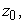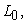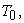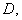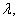and the reference value ofaccording to Equation (7). The error of the theoretical value ofcan be calculated from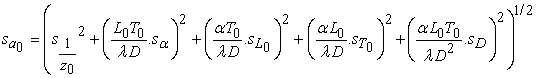(10)

where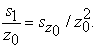### 3. Materials and Method

A photograph of the measurement system is shown in Figure 2. The metal rod to be investigated was held using a metal clamp and submerged in a water bath containing an electrical heating element. To reduce heat flow from the heated metal rod to the razor blades, each end of the heated metal rod was connected to one of a pair of razor blades through a vertical metal pillar and a horizontal wooden rod. A variac was used to vary and stabilize the electrical current flowing through the heating element. A Presenter P–1000 laser pointer was used as a source of red light of 650 nm wavelength emitted to the diffraction slit. The temperature sensor was attached to the metal rod, and a LabPro interface read the temperature data as a function of time and sent them to a Logger Pro display on a laptop.

• Figure 2. Photograph of the measurement system: (a) a variac, (b) the metal rod investigated, (c) the diffracting single-slit, (d) temperature sensor, (e) Logger Pro display, (f) laser pointer, (g) LabPro interface

The metal rod initial length (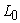) was 0.07 m and its width was 2.0 cm as measured by a 30.0 cm ruler, and the thickness was 0.5 mm as measured using a micrometer. The slit to screen distance, D was 2.38 m as measured by a tape ruler. The 30.0 cm plastic ruler was also used to measure 2z, i.e. the end-to-end width of the central bright line on the screen. In Figure 2, the screen is not visible.

Before heating the water, several minutes had to be allowed until the water temperature became constant at 30°C. The power supply for heating was turned on at the same time as the laser pointer, then the collect icon on Logger Pro was clicked. A graph of temperature (T) against time (t) would be displayed, and the distance between both ends of the central bright line, 2z was measured. For each metal, the data collection took 20 minutes for each run, and three runs were performed.

The weighted linear regression of the quantity 1/z against temperature was aided by a computational program which the author named REGLIN 9 to produce the linear coefficientsand(Equations 4.a and 4.b) as well as their errors (Equations 6.a and 6.b). Thereafter from Equation (9.a), the coefficient of linear thermal expansion,was calculated using the values of,D,while its error was calculated from Equation (9.b).

Besides a weighted linear regression, a goodness of fit test was also performed by the program to check whether the assumption of a linear relationagainst temperature is justified or not. For this purpose, the program also gave the reduced chi-square, defined as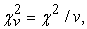where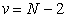is the degree of freedom, and P, the probability of obtaining a chi-square value from a random set of data which is larger or equal to the calculated chi-square. The set of data pairs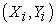, where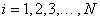is said to have a linear relation or the data set fit well to a linear function if P has a value in the range 10 - 90% 9.

### 4. Results and Discussion

Table 1 shows a summary of our experimental results for both metals, while Figure 3 shows graphs ofagainst temperature.

The second column in Table 1 shows values of P between 10-90% in all cases, which means that the theoretical linear relation betweenand temperature is fulfilled, as can be seen also in the graphs ofagainst temperature in Figure 3.

The third column in Table 1 presents the experimental values of the linear coefficientobtained from the weighted linear regression and their theoretical values as obtained from Equation (7) and their errors from Equation (10) using the reference value(s) ofshown in the last column in Table 1. For iron, the experimental value of a0=(5.91 ± 0.10) × 10m-1 and the theoretical value of a0=(6.04 ± 0.09) × 10m-1 agree well within limits of experimental errors. For brass, two slightly different reference values ofobtained by Inbanathan et al. using the optical lever method 7 and from a tabulated value 12 give theoretical values of a0=(4.75 ± 0.14) × 10m-1 and a0=(4.70 ± 0.14) × 10m-1, respectively. It can be seen that the experimental value of a0 = (4.50 ± 0.13) × 10m-1 obtained in this study agree well with both theoretical values.

• Figure 3. Graphs of 1/z against temperature for iron and brass

The last column in Table 1 shows the experimental coefficients of linear thermal expansión () obtained in this study and the reference values. For iron, this study gives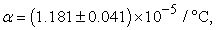in good agreement with the reference value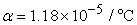12. For brass, this study gives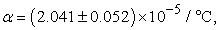slightly larger by 9.7% than the experimental value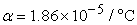7, and slightly larger by 9.4% than the accepted value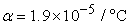12. The discrepancy between the experimental valuefor brass obtained in this study and these reference values 7, 12 can be attributed to the difference in composition since brass is an alloy containing copper and zinc at various proportions having values ofranging from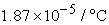to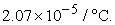The following limitations have been observed in the experimental design employed in this study. A series of experimental runs can not be performed quickly one after another because it needs about two hours to cool the apparatus to be ready for the next run. To improve the reliability of the results it would be desirable to have different initial metal rod lengthsbut this is difficult considering the small dimensions of the water bath. Furthermore, the observation of diffractions of higher orders is not possible since the lines on the screen will be diffuse.

It should also be noted that, by inspecting Equation (7), it is in principle also possible to obtainusing the values ofandbut then the relative errors inwill be much larger than those which will be obtained using our method as outlined in Section 3.

### 5. Conclusions

To summarize, a set of experiments using Logger Pro-aided observation of a single-slit diffraction pattern have been performed to determine the coefficients of linear thermal expansion of iron and brass. The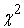goodness of fit test shows that the relation betweenand temperature is linear, in agreement with theory. The coefficient of linear thermal expansion for iron obtained agrees well with the reference value, while that for brass is close to the reference values.

### References

  Goodwin, A. L. “Nanomaterials: The ins and outs of thermal expansión,” Nature Nanotechnology, 3(12), 710-711, 2008. In article View Article  PubMed  Bedialauneta, M.T., Albizu, I., Fernandez, E. and Mazon, A. J., “Uncertainties in the testing of the coefficient of thermal expansion of overhead conductors,“ Energies, 13, 441-453, 2020. In article View Article  Jikihara, A. N., Tanaka, C. B. and Ballester, R. Y., “Why a zero CTE mismatch may be better for veneered Y-TZP structures?”, Journal of the Mechanical Behavior of Biomedical Materials, 96, 2019. In article View Article  PubMed  Lin, K., Yu, R., Wu, H. , Wang, F., Fang, L., Rong, Y., Huang, Q., Chen, J. and Xing, X., “Structure and thermal expansion of the tungsten bronze Pb2KNb5O15,” Dalton Transactions, 43, 7037-7043, 2014. In article View Article  PubMed  Kino, H., Fukushima, T. and Tanaka, T., “Remarkable suppression of local stress in 3D IC by manganese nitride-based filler with large negative CTE”, in IEEE 67th Electronic Components and Technology Conference (ECTC), May 2017. In article View Article  Fakhruddin, H., “Quantitative investigation of thermal expansion using single-slit diffraction,” Physics Teacher, 44, 82-84, 2006. In article View Article  Inbanathan, S. S. R., Moorthy, K. and Balasubramanian, G., “Measurement and demonstration of thermal expansion coefficient,” Physics Teacher, 45, 566-567, 2007. In article View Article  Joysey, W. D. and Mellinger, A., “Low-cost ultrasonic distance measurement in a mechanical resonance experiment”, preprint arXiv:1906.08778v1, 20 Jun 2019. In article  Oktova, R., “Tracker-aided experiments for the determination of viscosity coefficients of liquids using the communicating vessels method with weighted regression analysis,” Turkish Journal of Physics, 43, 383-392, 2019. In article  Bevington, P. R. and Robinson, D. K., Data Reduction and Error Analysis for the Physical Sciences, New York, USA: McGraw-Hill, 2003, 98-114. In article  Lide, D. R. (editor), CRC Handbook of Chemistry and Physics, 88th ed., Boca Raton, USA: Taylor & Francis, 2008, 12-200. In article  Agilent Laser and Optics User's Manual, Chapter 17 Material Expansion Coefficients, Agilent Technologies, 2002, 17-4. In articleThis work is licensed under a Creative Commons Attribution 4.0 International License. To view a copy of this license, visit http://creativecommons.org/licenses/by/4.0/